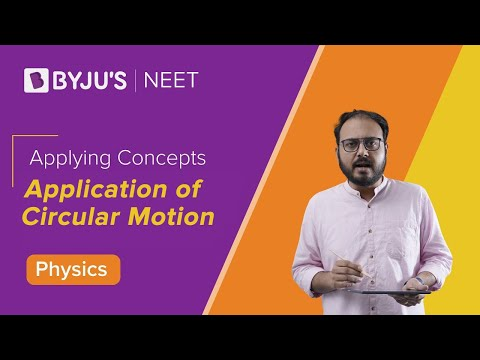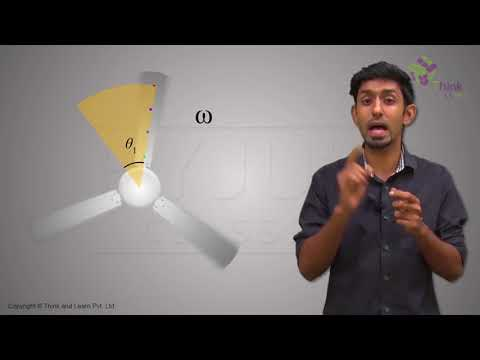# Uniform Circular Motion MCQs for NEET

The motion of the body moving with constant speed along a circular path is called Uniform Circular Motion. If the particle is moving in a circle, it must have some acceleration acting towards the centre which is making it move around the centre.So if a particle is moving in a uniform circular motion:

1) Its speed is constant

2) Velocity is changing at every instant

3) There is no tangential acceleration

4) Radial (centripetal) acceleration = ω2r,ω is the angular velocity and r is the radius of the circle.

Q1: The angular velocity of a body moving with a constant speed v in a circle of radius r is given by

1. v2/r
2. vr
3. v/r
4. r/v

Q2: A cyclist turns around a curve at 15 miles/hour. If he turns at double the speed, the tendency to overturn is

1. Doubled
3. Halved
4. Unchanged

Q3: What will be the velocity vector of a particle moving in a circle describing equal angles in equal times

1. Changes in direction
2. Remains constant
3. Changes in magnitude
4. Changes both in direction and magnitude

Q4: A body moves with a constant speed in a circular path is moving in a circular path with a constant speed. It has

1. Acceleration of constant magnitude
2. Constant velocity
3. Constant acceleration
4. An acceleration which varies with time

Answer: (a) Acceleration of constant magnitude

Q5: A motorcyclist going with a constant speed in a circular track has

1. Linear velocity is constant
2. Constant acceleration
3. Angular velocity is constant
4. Constant force

Answer: (c) Angular velocity is constant

Q6: What could be the reason a car moving on a horizontal road gets thrown out of the road while taking a turn

1. Due to the reaction of the ground
2. Due to rolling frictional force between tyre and road
3. By the gravitational force
4. Due to lack of sufficient centripetal force

Answer: (d) Due to lack of sufficient centripetal force

Q7: If a particle moves with constant angular velocity in a circle then during the motion its

1. Momentum is conserved
2. Energy is conserved
3. Both energy and momentum is conserved
4. None of the above is conserved

Q8: A stone tied to a string is rotated in a circle. If the string is cut, the stone flies away from the circle because

1. A centrifugal force acts on the stone
2. Of its inertia
3. A centripetal force acts on the stone
4. The reaction of the centripetal force

Q9: A body moves with a constant speed along a circle. Then,

1. No work is done on it
2. There is no acceleration produced in the body
3. No force acts on the body
4. The body has constant velocity

Answer: (a) No work is done on it

Q10: A tachometer is a device to measure

1. Gravitational pull
2. Speed of rotation
3. Surface tension
4. Tension in a spring